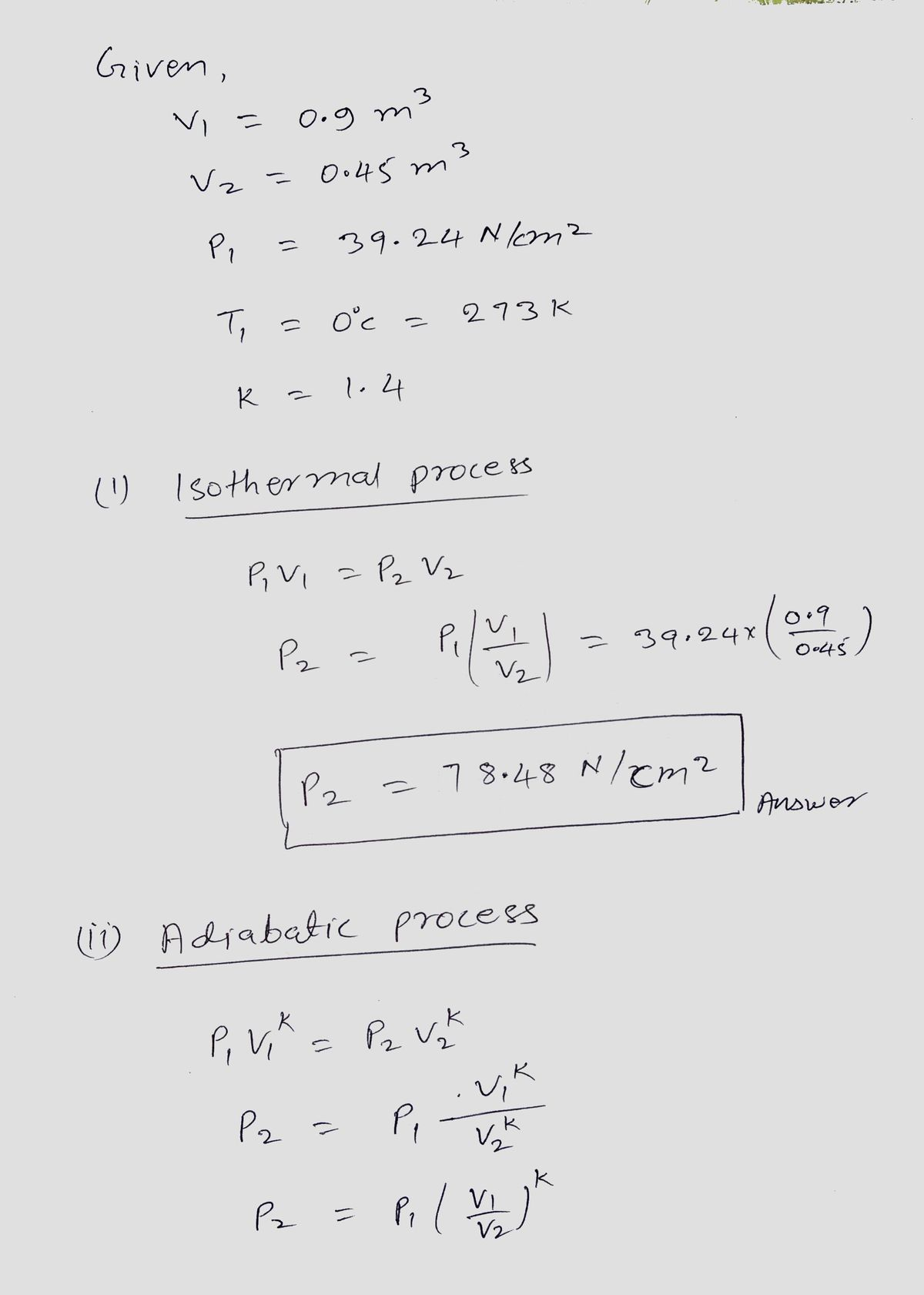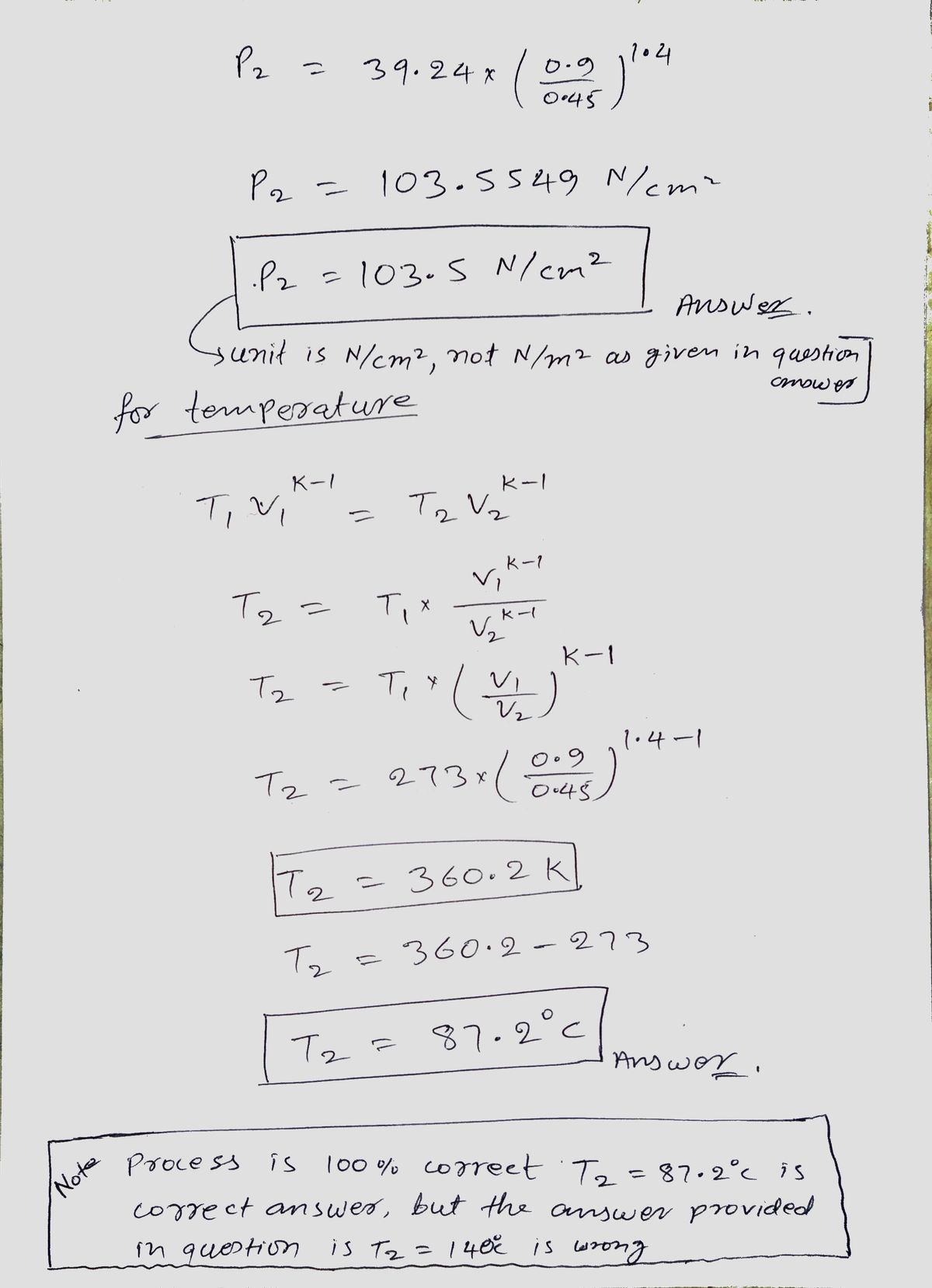# Calculate the pressure exerted by 4 kg mass of nitrogen gas at a temperature of 15°C if the volume is 0.35 m^3. Molecular weight of nitrogen is 28. 19.

Question-AnswerCategory: Fluid MechanicsCalculate the pressure exerted by 4 kg mass of nitrogen gas at a temperature of 15°C if the volume is 0.35 m^3. Molecular weight of nitrogen is 28. 19.

### Calculate the pressure exerted by 4 kg mass of nitrogen gas at a temperature of 15°C if the volume is 0.35 m^3. Molecular weight of nitrogen is 28. 19.